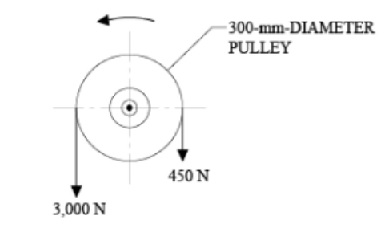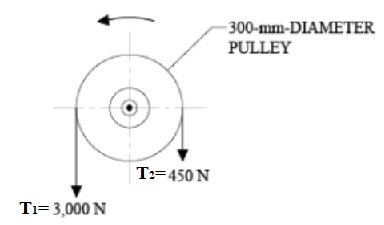# A pulley is driven by a belt as shown in the figure below. Neglecting centrifugal effects the...

## Question:

A pulley is driven by a belt as shown in the figure below. Neglecting centrifugal effects the minimum coefficient of friction that will prevent slipping between the belt and the pulley is most nearly:## Frictional Force:

It the force resisting the motion of a body when certain force is applied to that. The frictional force always acts in the opposite direction of the motion of the body.

Frictional force is the product of the coefficient of friction and the normal reaction (N).

{eq}F=\mu N {/eq}

Become a Study.com member to unlock this answer! Create your accountThe driving tension ratio is given by,

{eq}\dfrac {T_1}{T_2}=e^{\mu \theta } {/eq}

Where, {eq}T_1 {/eq} and {eq}T_2 {/eq} are the tensions...What is Friction? - Definition, Formula & Forces

from

Chapter 11 / Lesson 22
260K

Friction is the force that opposes the motion of an object. Learn about the sources of friction, identify examples of friction and test your knowledge with quiz questions.